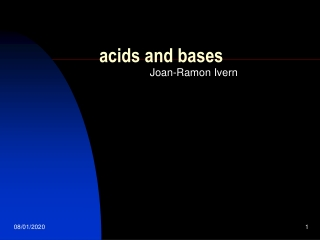Download Presentationacids and bases

# acids and bases

Download Presentation## acids and bases

- - - - - - - - - - - - - - - - - - - - - - - - - - - E N D - - - - - - - - - - - - - - - - - - - - - - - - - - -
##### Presentation Transcript

1. acids and bases Joan-Ramon Ivern

2. Acids One important group of chemicals is called acids. • Have you ever heard this word? Acid • When? Where? What do they do?

3. Some ideas • - stomach acidity • - lemon juice • - vinegar • - the liquid of a battery

4. - The acids in the laboratory • o Hydrochloric acid HCl • o Nitric acid HNO3 • o Sulphuric acid H2SO4 • o Ethanoic acid CH3COOH • o Citric acid

5. They are all liquids. In fact they are solutions of pure compounds in water. • Most of them must be handled carefully, especially the concentrated acids, because they are corrosive. They can eat away metals, skin and cloth.

6. But some acids are not so corrosive, they are the weak acids. • - Ethanoic acid (acetic acid). It’s found in vinegar. • - Citric acid. It’s found in lemon juice. • - Carbonic acid. It’s found in gaseous drinks

7. You can know if something is acid by its effect on litmus. It can be used as a solution or on paper. • If it turns red the substance is an acid.

8. Bases • What do we do against stomach acidity? • - We take baking soda, bicarbonate of soda, sodium hydrogen carbonate. • What do we do against a bee sting? • - We rub on ammonia lotion or calamine lotion which contains zinc carbonate. • Ant stings and nettle stings contain methanoic acid.

9. Any substance that neutralize an acid is called a base. • The sodium hydrogen carbonate, zinc carbonate, ammonia are bases. • This group of chemicals also affects litmus, but in a different way to acids. They turn litmus blue.

10. What causes acidity? • What makes that acids act alike are hydrogen ions. • Acids are solutions of pure compounds in water. The pure compounds are molecular. But in water, the molecules break up to form ions. They always give positive hydrogen ions. For example, in hydrochloric acid: • HCl(aq)  H+(aq) + Cl-(aq)

11. Bases are substances that remove H+ ions of the solution. • B(aq) + H+(aq)  BH+(aq)

12. The molecules of water (one into 555 milions) also break off giving H+ ions and OH- ions in equal number. It is said that water is neutral: • H2O  H+(aq) + OH-(aq)

13. The pH scale • The strength of an acid is measured in a scale of numbers called the pH scale. The numbers go from 0 to 14. • Pure water is neutral and has a pH of 7 in the middle of the pH scale.

14. Strong acids leave a great number of H+(aq) ions. • Weak acids leave a little number of H+(aq) ions. • The numbers from 0 to 7 correspond to acids. The less is the number stronger is the acid.

15. A solution of a base in water will remove some H+ ions and its concentration will decrease and the pH will be bigger than 7. • B(aq) + H+(aq) à BH+(aq) • The strength of bases increases from 7 to 14 in the pH scale.

16. You can find the pH of any solution by using universal indicator. • Universal indicator is a mixture of dyes. • It goes a different colour at different pH, from • red (pH = 0), • yellow pH = 4-5, • green (pH = 7), • greenish-blue (pH = 8-9), • blue (pH = 10-11), • violet (pH = 12-14).

17. The solutions with a pH between 7 and 14 are called alkaline solutions. • And an important group of bases receive the name of alkalis.

18. Most pure alkalis are solids. But they are usually used in laboratory as aqueous solutions. The main ones are: • - sodium hydroxide NaOH(aq) • - potassium hydroxide KOH(aq) • - calcium hydroxide Ca(OH)2(aq) • - ammonia NH3(aq). Pure NH3 is a gas.

19. A little of history of the definition of ACID and BASE. • First of all we can see that the ion H+ is the nucleus of the hydrogen atom, that's a proton. • In water solution a proton, H+, cannot exist in isolation and is solvated. In water it exists as H3O+. • The dissociation of ethanoic acid (acetic acid) should be written as: • CH3COOH + H2O  CH3COO- + H3O+

20. However, for convenience, we will usually write it as: • CH3COOH <----> CH3COO- + H+ • Similarly, for bases • :NH3 + H2O = NH4+ + HO- • will usually be written as • :NH3 + H+  NH4+

21. Arrehnius defined: ACID as a substance that leaves H+ ions in water solution. • HA(aq)  H+(aq) + A-(aq) • BASE as a substance that leaves OH- ions in water solution. • BOH  B+ + OH-

22. Broensted and Lorry defined: • ACID as a substance that leaves H+ ions in water solution. It is a proton donor. • HA H++  A- • BASE as a substance that removes H+ ions from water solution. It is a proton acceptor. • B+ H+ BH+

23. Lewis defined: • ACID as a substance that accepts an electron pair. It's an electron pair acceptor. • BASE as a substance has an electron pair to donate. It's an electron pair donor. • :NH3 + H+ = NH4+ Base acid